# 专栏 | 手机端运行卷积神经网络实践：基于TensorFlow和OpenCV实现文档检测功能

2017年05月30日 13:45 机器之心

1. 前言

2. 需求是什么

3. 传统的技术方案

4. 传统技术方案的难度和局限性

5. 思考如何改善

6. 无效的神经网络算法

7. 有效的神经网络算法

8. 训练网络

9. 训练数据集 (大量合成数据 + 少量真实数据)

10. 在手机设备上运行 TensorFlow

11. HED 网络在手机上遇到的奇怪 crash

12. 裁剪 TensorFlow

13. 裁剪 HED 网络

14. TensorFlow API 的选择

15. OpenCV 算法

16. 总结

17. 参考文献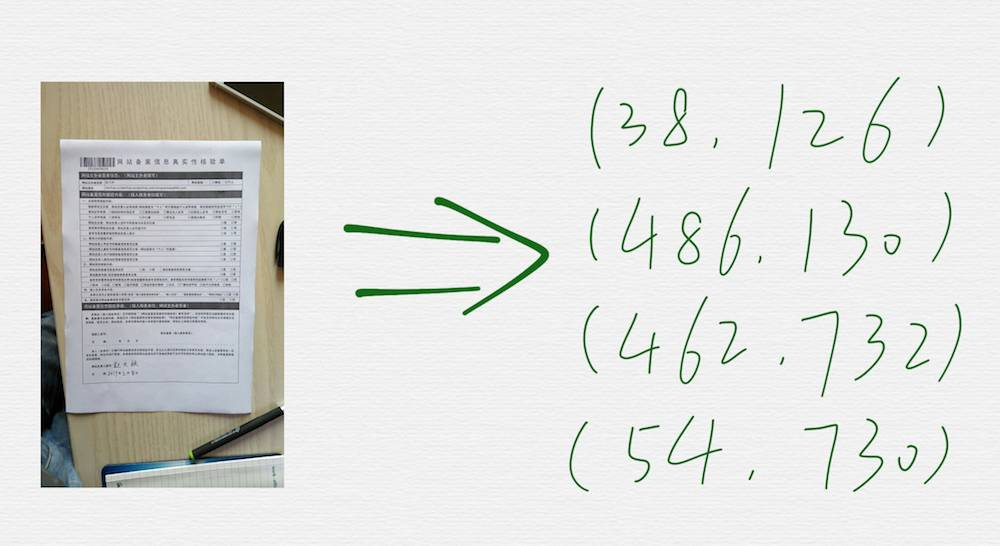Google 搜索 opencv scan document，是可以找到好几篇相关的教程的，这些教程里面的技术手段，也都大同小异，关键步骤就是调用 OpenCV 里面的两个函数，cv2.Canny() 和 cv2.findContours()。canny 算法的检测效果，依赖于几个阀值参数，这些阀值参数的选择，通常都是人为设置的经验值，在改进的过程中，引入额外的步骤后，通常又会引入一些新的阀值参数，同样，也是依赖于调试结果设置的经验值。整体来看，这些阀值参数的个数，不能特别的多，因为一旦太多了，就很难依赖经验值进行设置，另外，虽然有这些阀值参数，但是最终的参数只是一组或少数几组固定的组合，所以算法的鲁棒性又会打折扣，很容易遇到边缘检测效果不理想的场景end-to-end 直接拟合

face alignment 之所以可以用回归网络得到很好的拟合效果，是因为在输入 image 上先做了 bounding box 检测，缩小了人脸图像范围后，才做的 regression

YOLO && FCNHED(Holistically-Nested Edge Detection) 网络

HED 网络模型是在 VGG16 网络结构的基础上设计出来的，所以有必要先看看 VGG16。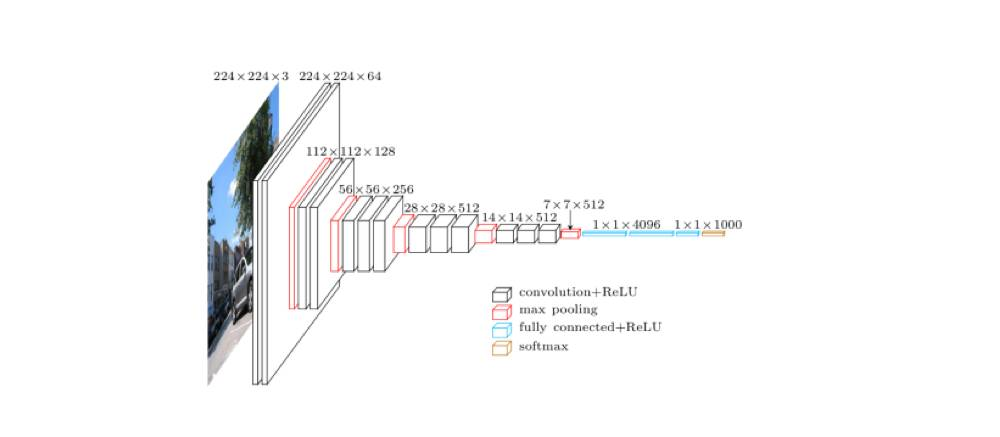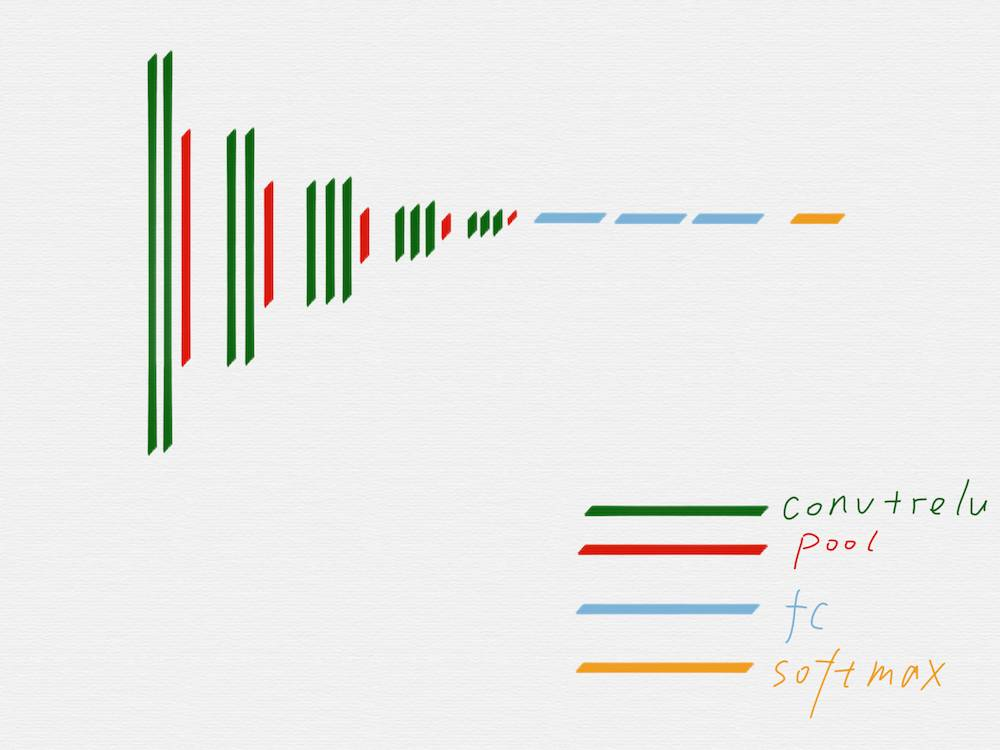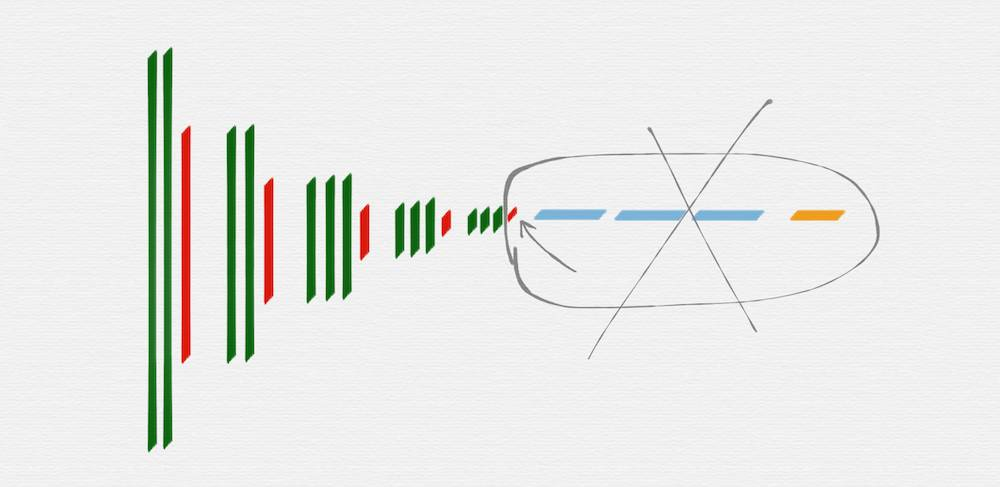HED 网络要使用的就是 VGG16 网络里面的这五组，后面部分的 fully connected 层和 softmax 层，都是不需要的，另外，第五组的池化层 (红色) 也是不需要的。HED 网络是一种多尺度多融合 (multi-scale and multi-level feature learning) 的网络结构，所谓的多尺度，就是如上图所示，把 VGG16 的每一组的最后一个卷积层 (绿色部分) 的输出取出来，因为每一组得到的 image 的长宽尺寸是不一样的，所以这里还需要用转置卷积 (transposed convolution)/ 反卷积 (deconv) 对每一组得到的 image 再做一遍运算，从效果上看，相当于把第二至五组得到的 image 的长宽尺寸分别扩大 2 至 16 倍，这样在每个尺度 (VGG16 的每一组就是一个尺度) 上得到的 image，都是相同的大小了。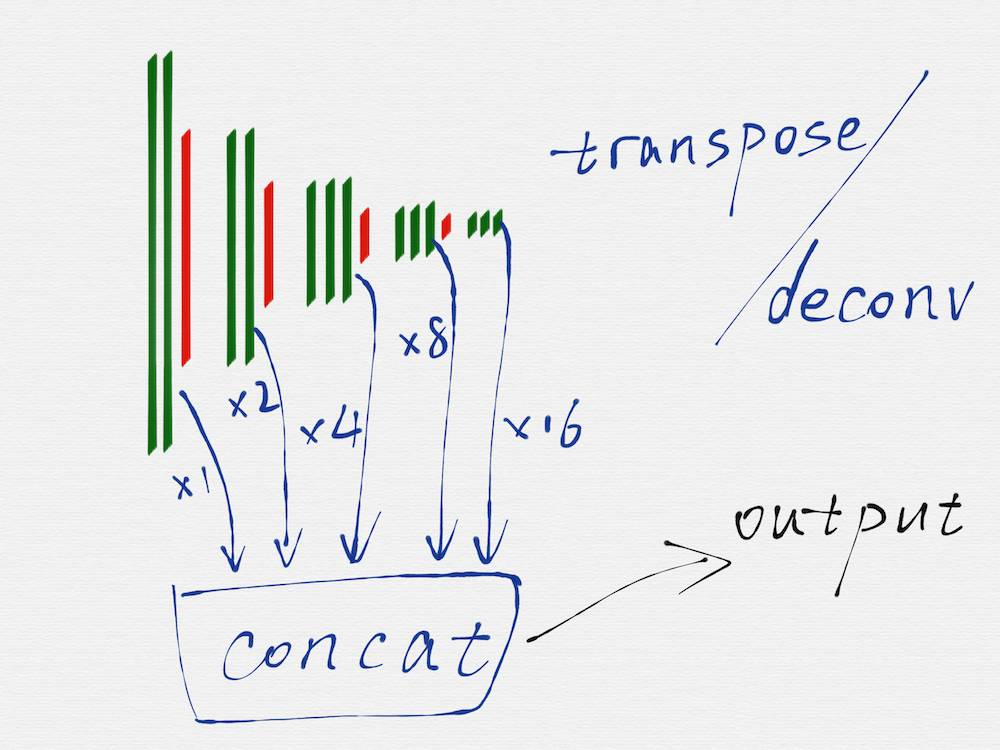def hed_net(inputs, batch_size):

# ref https://github.com/s9xie/hed/blob/master/examples/hed/train_val.prototxt

with tf.variable_scope('hed', 'hed', [inputs]):

with slim.arg_scope([slim.conv2d, slim.fully_connected],

activation_fn=tf.nn.relu,

weights_initializer=tf.truncated_normal_initializer(0.0, 0.01),

weights_regularizer=slim.l2_regularizer(0.0005)):

# vgg16 conv && max_pool layers

net = slim.repeat(inputs, 2, slim.conv2d, 12, [3, 3], scope='conv1')

dsn1 = net

net = slim.max_pool2d(net, [2, 2], scope='pool1')

net = slim.repeat(net, 2, slim.conv2d, 24, [3, 3], scope='conv2')

dsn2 = net

net = slim.max_pool2d(net, [2, 2], scope='pool2')

net = slim.repeat(net, 3, slim.conv2d, 48, [3, 3], scope='conv3')

dsn3 = net

net = slim.max_pool2d(net, [2, 2], scope='pool3')

net = slim.repeat(net, 3, slim.conv2d, 96, [3, 3], scope='conv4')

dsn4 = net

net = slim.max_pool2d(net, [2, 2], scope='pool4')

net = slim.repeat(net, 3, slim.conv2d, 192, [3, 3], scope='conv5')

dsn5 = net

# net = slim.max_pool2d(net, [2, 2], scope='pool5') # no need this pool layer

# dsn layers

dsn1 = slim.conv2d(dsn1, 1, [1, 1], scope='dsn1')

# no need deconv for dsn1

dsn2 = slim.conv2d(dsn2, 1, [1, 1], scope='dsn2')

deconv_shape = tf.pack([batch_size, const.image_height, const.image_width, 1])

dsn2 = deconv_mobile_version(dsn2, 2, deconv_shape) # deconv_mobile_version can work on mobile

dsn3 = slim.conv2d(dsn3, 1, [1, 1], scope='dsn3')

deconv_shape = tf.pack([batch_size, const.image_height, const.image_width, 1])

dsn3 = deconv_mobile_version(dsn3, 4, deconv_shape)

dsn4 = slim.conv2d(dsn4, 1, [1, 1], scope='dsn4')

deconv_shape = tf.pack([batch_size, const.image_height, const.image_width, 1])

dsn4 = deconv_mobile_version(dsn4, 8, deconv_shape)

dsn5 = slim.conv2d(dsn5, 1, [1, 1], scope='dsn5')

deconv_shape = tf.pack([batch_size, const.image_height, const.image_width, 1])

dsn5 = deconv_mobile_version(dsn5, 16, deconv_shape)

# dsn fuse

dsn_fuse = tf.concat(3, [dsn1, dsn2, dsn3, dsn4, dsn5])

dsn_fuse = tf.reshape(dsn_fuse, [batch_size, const.image_height, const.image_width, 5]) #without this, will get error: ValueError: Number of in_channels must be known.

dsn_fuse = slim.conv2d(dsn_fuse, 1, [1, 1], scope='dsn_fuse')

return dsn_fuse, dsn1, dsn2, dsn3, dsn4, dsn5

cost 函数

input_queue_for_train = tf.train.string_input_producer([FLAGS.csv_path])

image_tensor, annotation_tensor = input_image_pipeline(dataset_root_dir_string, input_queue_for_train, FLAGS.batch_size)

dsn_fuse, dsn1, dsn2, dsn3, dsn4, dsn5 = hed_net(image_tensor, FLAGS.batch_size)

cost = class_balanced_sigmoid_cross_entropy(dsn_fuse, annotation_tensor) + \

class_balanced_sigmoid_cross_entropy(dsn1, annotation_tensor) + \

class_balanced_sigmoid_cross_entropy(dsn2, annotation_tensor) + \

class_balanced_sigmoid_cross_entropy(dsn3, annotation_tensor) + \

class_balanced_sigmoid_cross_entropy(dsn4, annotation_tensor) + \

class_balanced_sigmoid_cross_entropy(dsn5, annotation_tensor)

input_queue_for_train = tf.train.string_input_producer([FLAGS.csv_path])

image_tensor, annotation_tensor = input_image_pipeline(dataset_root_dir_string, input_queue_for_train, FLAGS.batch_size)

dsn_fuse, _, _, _, _, _ = hed_net(image_tensor, FLAGS.batch_size)

cost = class_balanced_sigmoid_cross_entropy(dsn_fuse, annotation_tensor)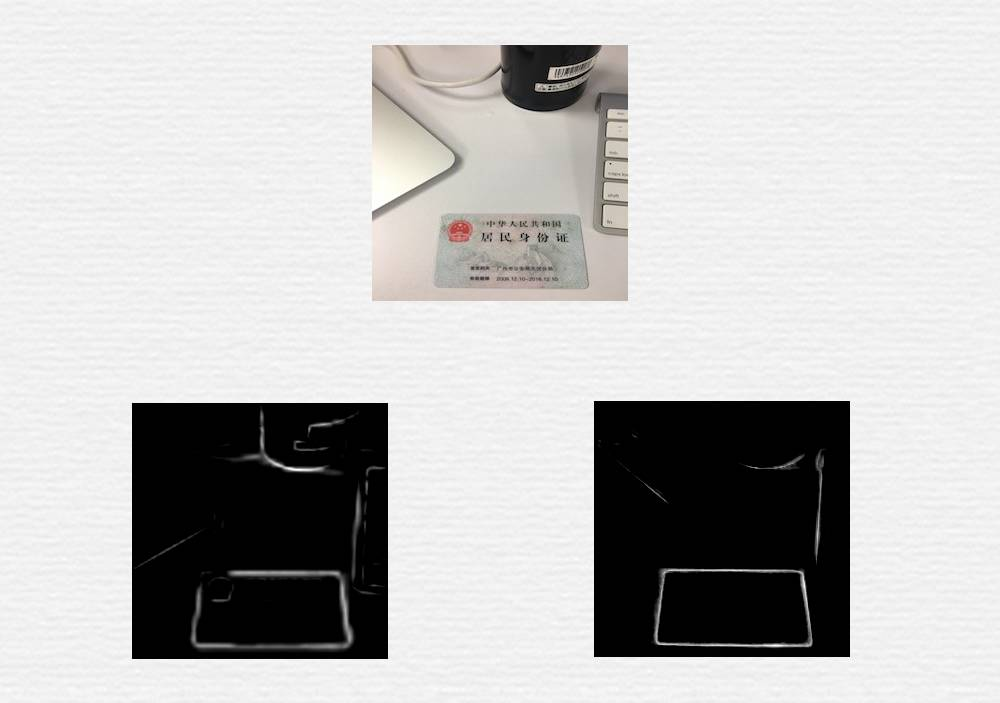def class_balanced_sigmoid_cross_entropy(logits, label, name='cross_entropy_loss'):

"""

The class-balanced cross entropy loss,

as in `Holistically-Nested Edge Detection

<http://arxiv.org/abs/1504.06375>`_.

This is more numerically stable than class_balanced_cross_entropy

:param logits: size: the logits.

:param label: size: the ground truth in {0,1}, of the same shape as logits.

:returns: a scalar. class-balanced cross entropy loss

"""

y = tf.cast(label, tf.float32)

count_neg = tf.reduce_sum(1. - y) # the number of 0 in y

count_pos = tf.reduce_sum(y) # the number of 1 in y (less than count_neg)

beta = count_neg / (count_neg + count_pos)

pos_weight = beta / (1 - beta)

cost = tf.nn.weighted_cross_entropy_with_logits(logits, y, pos_weight)

cost = tf.reduce_mean(cost * (1 - beta), name=name)

return cost

HED 的论文中，并没有明确的要求也要采用这种方式初始化转置卷积层，但是，在训练过程中发现，采用这种方式进行初始化，模型才更容易收敛。

def get_kernel_size(factor):

"""

Find the kernel size given the desired factor of upsampling.

"""

return 2 * factor - factor % 2

def upsample_filt(size):

"""

Make a 2D bilinear kernel suitable for upsampling of the given (h, w) size.

"""

factor = (size + 1) // 2

if size % 2 == 1:

center = factor - 1

else:

center = factor - 0.5

og = np.ogrid[:size, :size]

return (1 - abs(og - center) / factor) * (1 - abs(og - center) / factor)

def bilinear_upsample_weights(factor, number_of_classes):

"""

Create weights matrix for transposed convolution with bilinear filter

initialization.

"""

filter_size = get_kernel_size(factor)

weights = np.zeros((filter_size,

filter_size,

number_of_classes,

number_of_classes), dtype=np.float32)

upsample_kernel = upsample_filt(filter_size)

for i in xrange(number_of_classes):

weights[:, :, i, i] = upsample_kernel

return weights

HED 网络不像 VGG 网络那样很容易就进入收敛状态，也不太容易进入期望的理想状态，主要是两方面的原因：

HED 论文里使用的训练数据集，是针对通用的边缘检测目的的，什么形状的边缘都有，比如下面这种：OpenCV 不支持透明图层之间的旋转和透视变换操作，只能使用最低精度的插值算法，为了改善这一点，后续改成了使用 iOS 模拟器，通过 CALayer 上的操作来合成图片TensorFlow 官方是支持 iOS 和 Android 的，而且有清晰的文档，照着做就行。但是因为 TensorFlow 是依赖于 protobuf 3 的，所以有可能会遇到一些其他的问题，比如下面这两种，就是我们在两个不同的 iOS APP 中遇到的问题和解决办法，可以作为一个参考：

A 产品使用的是 protobuf 2，同时由于各种历史原因，使用并且停留在了很旧的某个版本的 Base 库上，而 protobuf 3 的内部也使用了 Base 库，当 A 产品升级到 protobuf 3 后，protobuf 3 的 Base 库和 A 源码中的 Base 库产生了一些奇怪的冲突，最后的解决办法是手动修改了 A 源码中的 Base 库，避免编译时的冲突

B 产品也是使用的 protobuf 2，而且 B 产品使用到的多个第三方模块 (没有源码，只有二进制文件) 也是依赖于 protobuf 2，直接升级 B 产品使用的 protobuf 库就行不通了，最后采用的方法是修改 TensorFlow 和 TensorFlow 中使用的 protobuf 3 的源代码，把 protobuf 3 换了一个命名空间，这样两个不同版本的 protobuf 库就可以共存了

Android 上因为本身是可以使用动态库的，所以即便 app 必须使用 protobuf 2 也没有关系，不同的模块使用 dlopen 的方式加载各自需要的特定版本的库就可以了。

HED 网络在手机上遇到的奇怪 crash

Invalid argument: No OpKernel was registered to support Op 'Mul' with these attrs.  Registered devices: [CPU], Registered kernels:

device='CPU'; T in [DT_FLOAT]

[[Node: hed/mul_1 = Mul[T=DT_INT32](hed/strided_slice_2, hed/mul_1/y)]]

Google 搜索后发现很多人遇到过类似的情况，但是错误信息又并不相同，后来在 TensorFlow 的 github issues 里终于找到了线索，综合起来解释，是因为 TensorFlow 是基于操作 (Operation) 来模块化设计和编码的，每一个数学计算模块就是一个 Operation，由于各种原因，比如内存占用大小、GPU 独占操作等等，mobile 版的 TensorFlow，并没有包含所有的 Operation，mobile 版的 TensorFlow 支持的 Operation 只是 PC 完整版 TensorFlow 的一个子集，我遇到的这个错误，就是因为使用到的某个 Operation 并不支持 mobile 版。

def deconv(inputs, upsample_factor):

input_shape = tf.shape(inputs)

# Calculate the ouput size of the upsampled tensor

upsampled_shape = tf.pack([input_shape,

input_shape * upsample_factor,

input_shape * upsample_factor,

1])

upsample_filter_np = bilinear_upsample_weights(upsample_factor, 1)

upsample_filter_tensor = tf.constant(upsample_filter_np)

# Perform the upsampling

upsampled_inputs = tf.nn.conv2d_transpose(inputs, upsample_filter_tensor,

output_shape=upsampled_shape,

strides=[1, upsample_factor, upsample_factor, 1])

return upsampled_inputs

def deconv_mobile_version(inputs, upsample_factor, upsampled_shape):

upsample_filter_np = bilinear_upsample_weights(upsample_factor, 1)

upsample_filter_tensor = tf.constant(upsample_filter_np)

# Perform the upsampling

upsampled_inputs = tf.nn.conv2d_transpose(inputs, upsample_filter_tensor,

output_shape=upsampled_shape,

strides=[1, upsample_factor, upsample_factor, 1])

return upsampled_inputs

TensorFlow 是一个很庞大的框架，对于手机来说，它占用的体积是比较大的，所以需要尽量的缩减 TensorFlow 库占用的体积。

tensorflow/core/kernels/xent_op.cc

tensorflow/core/kernels/where_op.cc

tensorflow/core/kernels/unpack_op.cc

tensorflow/core/kernels/transpose_op.cc

tensorflow/core/kernels/transpose_functor_cpu.cc

tensorflow/core/kernels/tensor_array_ops.cc

tensorflow/core/kernels/tensor_array.cc

tensorflow/core/kernels/split_op.cc

tensorflow/core/kernels/split_v_op.cc

tensorflow/core/kernels/split_lib_cpu.cc

tensorflow/core/kernels/shape_ops.cc

tensorflow/core/kernels/session_ops.cc

tensorflow/core/kernels/sendrecv_ops.cc

tensorflow/core/kernels/reverse_op.cc

tensorflow/core/kernels/reshape_op.cc

tensorflow/core/kernels/relu_op.cc

tensorflow/core/kernels/pooling_ops_common.cc

tensorflow/core/kernels/pack_op.cc

tensorflow/core/kernels/ops_util.cc

tensorflow/core/kernels/no_op.cc

tensorflow/core/kernels/maxpooling_op.cc

tensorflow/core/kernels/matmul_op.cc

tensorflow/core/kernels/immutable_constant_op.cc

tensorflow/core/kernels/identity_op.cc

tensorflow/core/kernels/gather_op.cc

tensorflow/core/kernels/gather_functor.cc

tensorflow/core/kernels/fill_functor.cc

tensorflow/core/kernels/dense_update_ops.cc

tensorflow/core/kernels/deep_conv2d.cc

tensorflow/core/kernels/xsmm_conv2d.cc

tensorflow/core/kernels/conv_ops_using_gemm.cc

tensorflow/core/kernels/conv_ops_fused.cc

tensorflow/core/kernels/conv_ops.cc

tensorflow/core/kernels/constant_op.cc

tensorflow/core/kernels/concat_op.cc

tensorflow/core/kernels/concat_lib_cpu.cc

tensorflow/core/kernels/bias_op.cc

tensorflow/core/ops/sendrecv_ops.cc

tensorflow/core/ops/no_op.cc

tensorflow/core/ops/nn_ops.cc

tensorflow/core/ops/array_ops.cc

HED 网络是用 VGG16 作为基础网络结构，而 VGG 又是一个得到广泛验证的基础网络结构，因此修改 HED 的整体结构肯定不是一个明智的选择，至少不是首选的方案。

TensorFlow API 的选择

TensorFlow 的 API 是很灵活的，也比较底层，在学习过程中发现，每个人写出来的代码，风格差异很大，而且很多工程师又采用了各种各样的技巧来简化代码，但是这其实反而在无形中又增加了代码的阅读难度，也不利于代码的复用。

OpenCV 算法end-to-end 网络无效的时候，可以用 pipeline 的思路考虑问题、拆分业务，针对性的使用神经网络技术